Showing Page:
1/9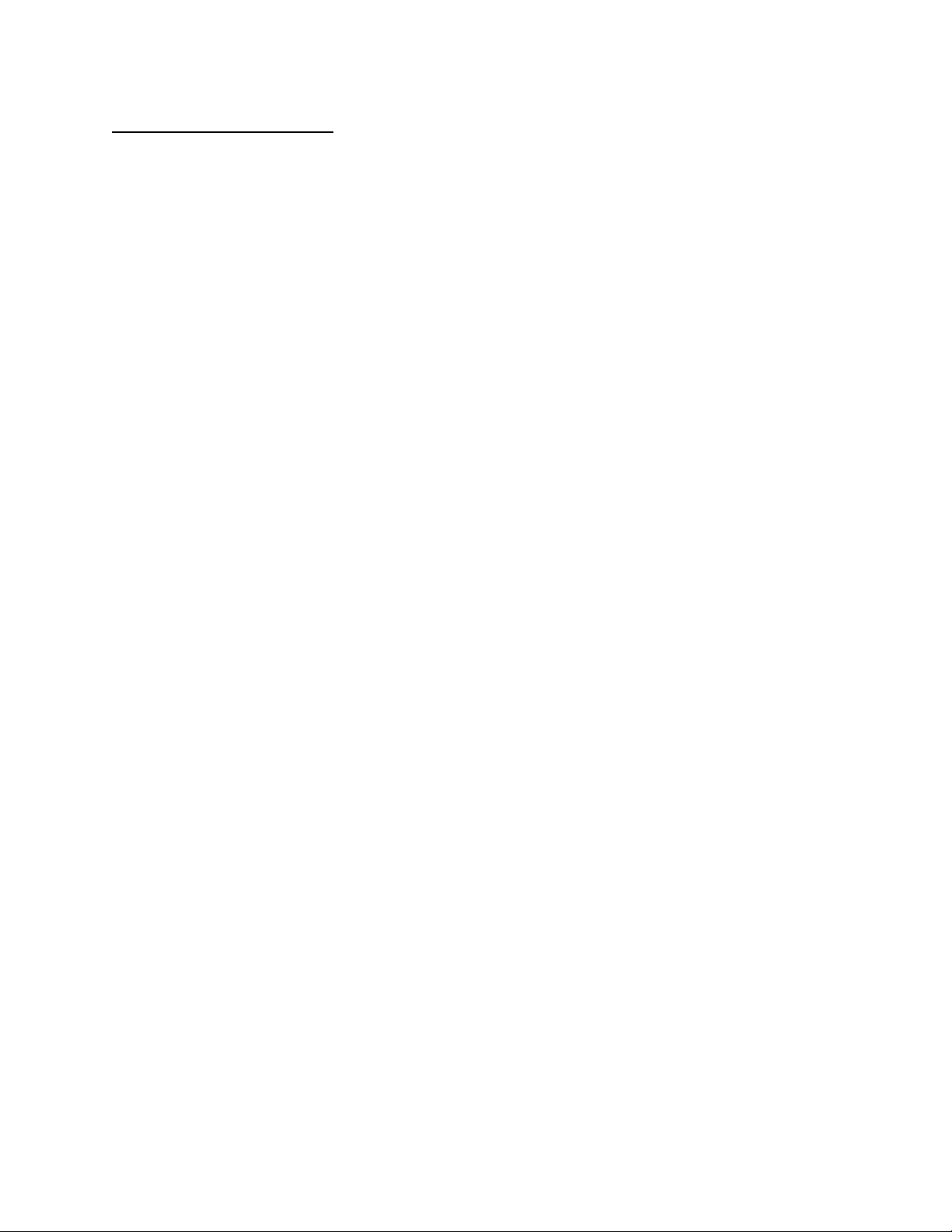REGRESSION ANALYSIS
. Regression analysis is concerned with the study of the dependence of one variable (the
Dependent variable) on one or more other variables (the Explanatory Variable(s) with a
view to estimating and/ or predicting the average value of the dependent variable.
Regression analysis aims at finding the equation of the line of best fit in relationship between
dependent variable and independent variables. The idea of the line of best fit is derived from
the fact that the relationship between Y and X may not be depicted by a straight line such
that all coordinates of X and Y falls on that line.
The line of best fit therefore is the line that passes through the points capturing the
relationship between dependent and independent variables the best way compared to any
other line.
Regression Analysis therefore enable us to come up with mathematical equation of this line
of best fit. In case there is linear relationship between X and Y, the equation of line of best
fit or regression line will be given as follows:
01
ˆ
Y b b X=+
, such that:
ˆ
Y
≡ refer to the estimated values of Y on the line of best fit for any value of X
X≡ explanatory variable whose values are usually assume to be fixed or given.
0
b
= intercept
1
b
Showing Page:
2/9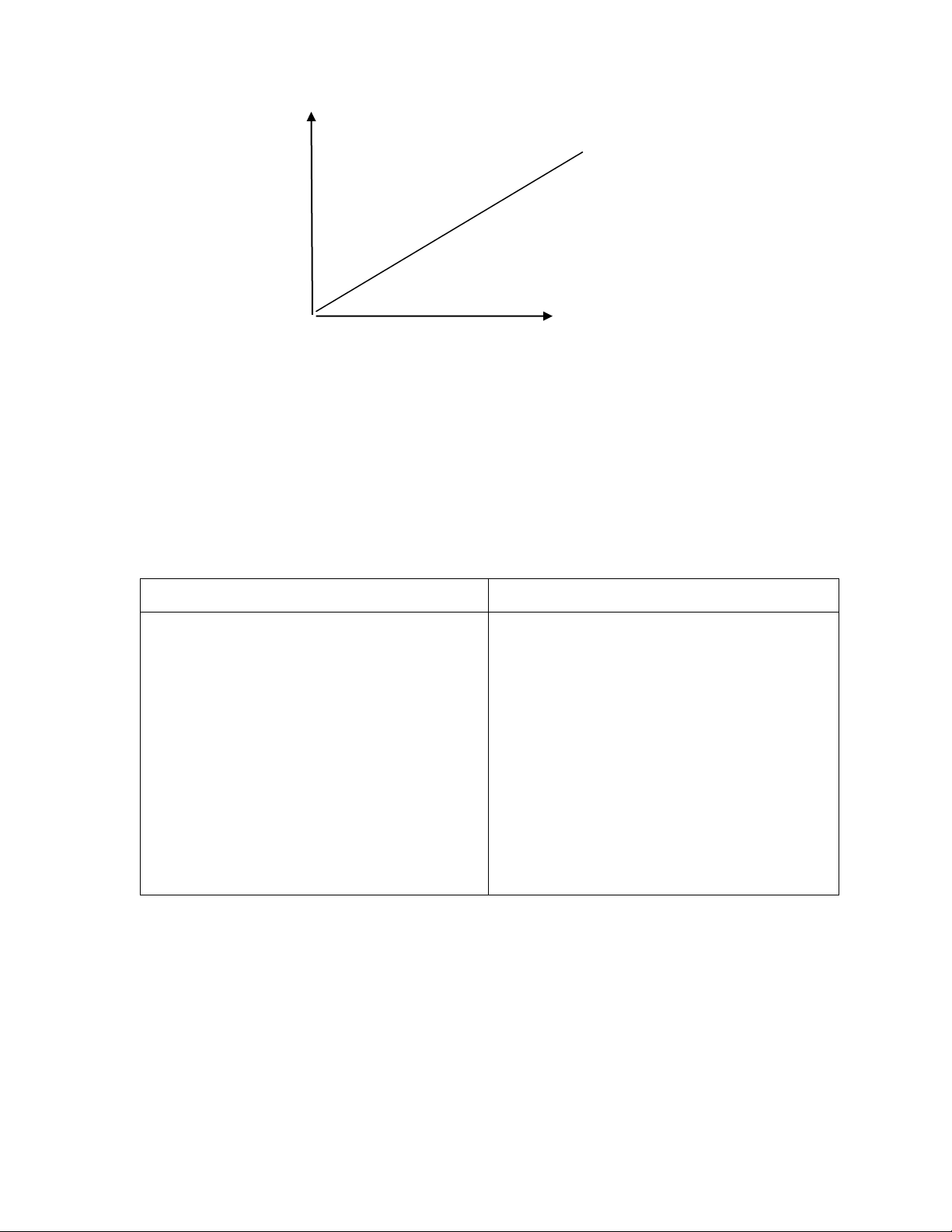Y . .
01
ˆ
ii
YX

=+
. . .
. . .
X
There are thus two (2) primary differences between correlation and regression analysis, as
outlined below:
CORRELATION
REGRESSION
i) Assumes symmetry between X
and Y i.e., there is no distinction
as to which variable is dependent
(causality is not important)
ii) Both X and Y are assumed to be
statistical, random or stochastic
i) Assumes asymmetry between X
and Y; i.e., distinguishes which
variable is dependent and which
is explanatory (causality is
important)
ii) Only Y is assumed to be
statistical but X is assumed to be
fixed.
Thus, correlation does not imply causality, but regression does so.
There are basically two types of regression analysis, i.e.,
i) Simple regression analysis
ii) Multiple regression analysis
Showing Page:
3/9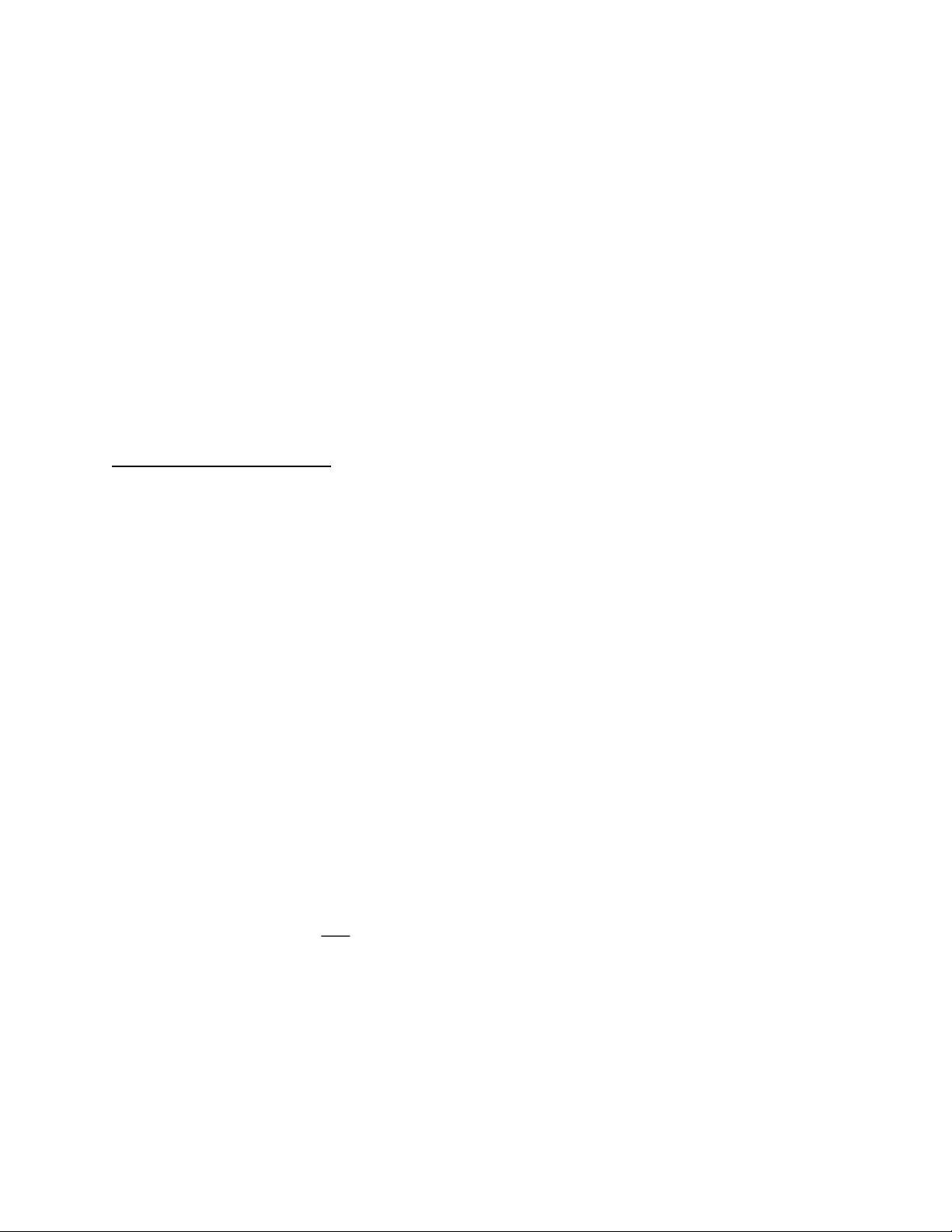In simple regression analysis, we study the effect of only one explanatory variable on the
dependent variable. For example, how X affects Y. Thus, Y=F(X)
For this reason, simple regression analysis is also known bivariate regression.
In multiple regression analysis, we study the effect of more than one explanatory variable
on the dependent variable. For example, how changes in X
1
, X2 and X
3
will affect Y in:
Thus Y=F(X
1
, X
2
, …………, Xn)
.
Simple Regression Analysis
In performing regression analysis, we can use either the population or a sample data.
The population regression model is given by
The sample regression is given by:
01ii
Y b b X e= + +
Where:
-
i
Y
is the actual value of dependent variable ( for the population or sample)
- X
i
is the independent variable
0
and
0
b
are the intercept terms of the population sample regression models
respectively.
1
and
1
b
are the slopes or partial derivative of the population regression models
respectively i.e.
11
i
i
Y
or b
X
=
as the case may be.
i
u
is the error term of the population regression model (
()
i
i
YY
i
e
is the error term of the sample regression model
()
i
i
YY
Showing Page:
4/9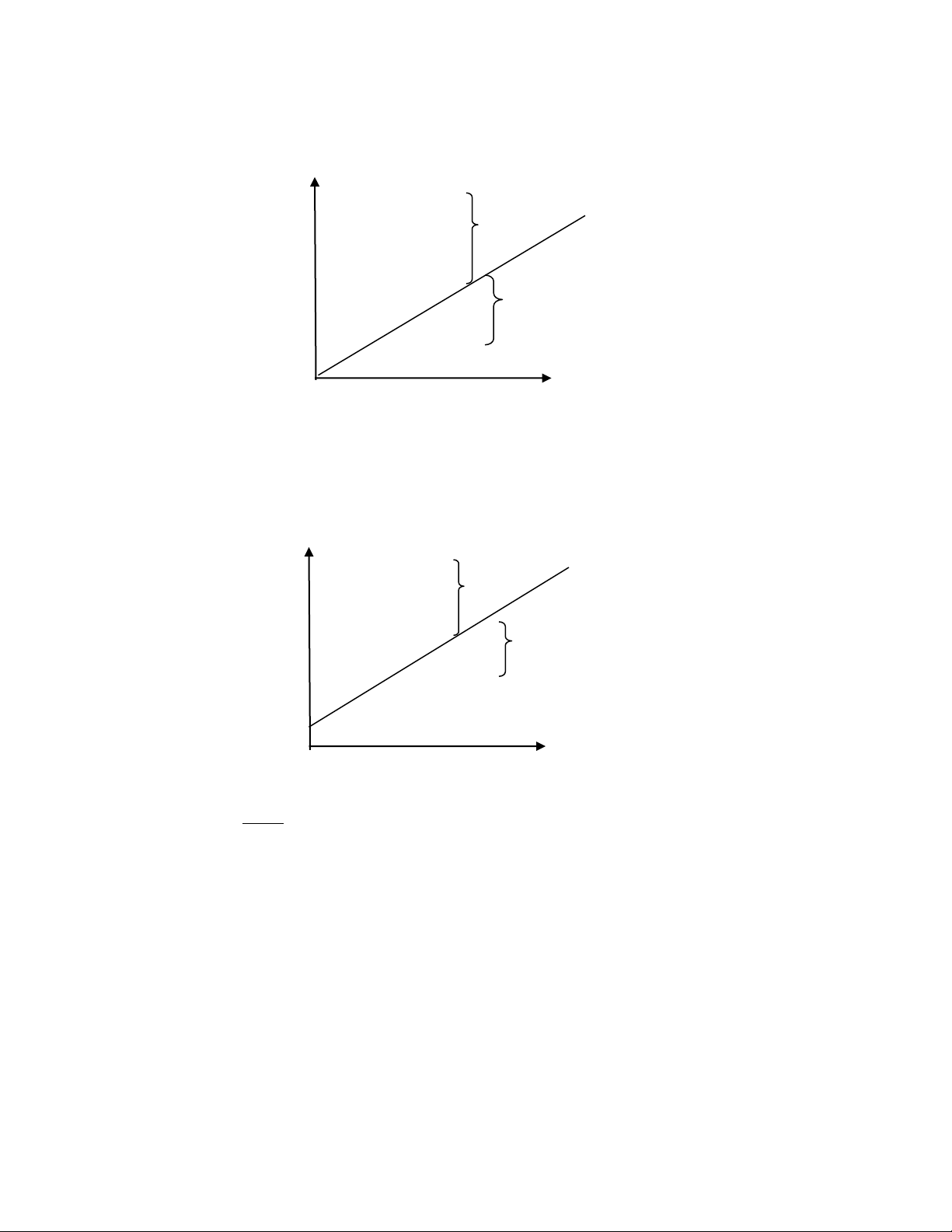Population Regression
.
Y . . .+U .
01
i
i
YX

=+
. . -U
. . .
.
. x
Sample Regression
y . . +e .
01
i
i
Y b b X
=+
. . . -e
. . . . . .
01i i i
Y b b X e= + +
. x
Thus, the error is the difference between each observation
Y
and the estimated value of Y
on the regression line (
Y
) for any given value of X
While
01
i
i
Y b b X
=+
is the equation of the line of best fit,
01
i
ii
Y b b X e= + +
give coordinate of
any point in the data
In regression analysis, we prefer using a sample rather than the population data. This is
because, in real life or practice, it is easier to observe a sample data as compared to
.
-
-
u
Showing Page:
5/9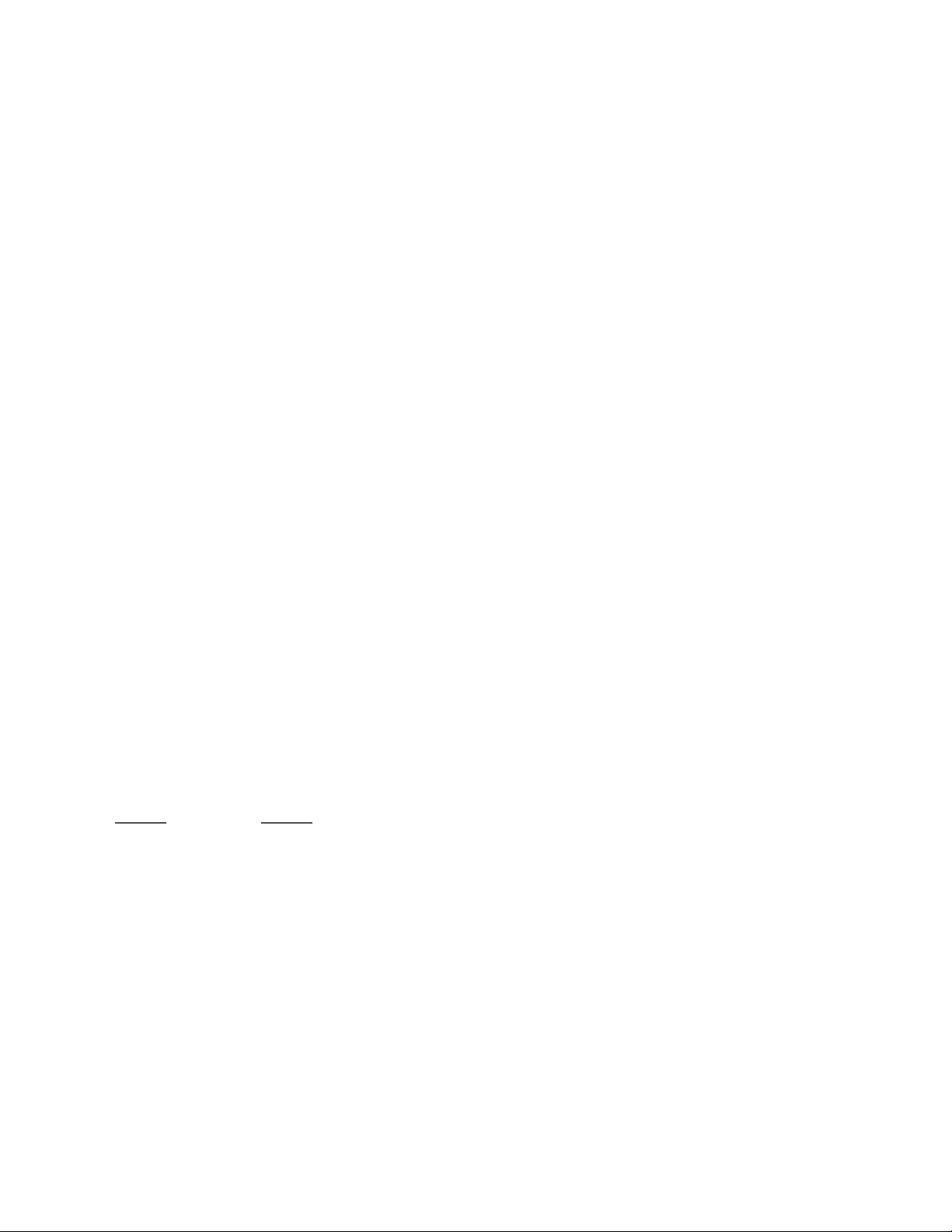observing population data. We then use sample regression intercept and slope
01
b and b
as
estimators of unknown population parameters (
01
and

)
The Ordinary Least Squares (OLS) Estimation
The Ordinary Least Squares (OLS) Estimation is the main techniques used to estimate
regression models/equations.
The name OLS is derived from the fact that this method aims at getting regression
coefficients
01
b and b
by minimizing the sum of squared errors.
To illustrate, consider the following sample regression model;
01i i i
Y b b X e= + +
From this model let’s make error (e
i)
to be the subject of the above equation as follows;
01i i i
e Y b b X=
OLS require that we square the error and then sum up all the errors in square form ie
( )
2
2
01
11
nn
i i i
ii
e Y b b X
==
=

The last step to get the estimates of
01
b and b
by minimizing
2
i
e
with respect to the
two coefficients. This is by getting partial derivative and then equate to zero
22
01
00
ii
ee
and
bb


==

. We then solve the resulting two equations simultaneously. The
resulting values of
01
b and b
are the one that correspond to the line of best fit.
The ordinary least squares estimators (
0
b
and
1
b
) are called so because they are used as
estimators of unknown population regression parameters
0
B
and
1
B
.
As noted above OLS technique has several steps but in summary these two estimators are
derived use the following formulas:
Showing Page:
6/9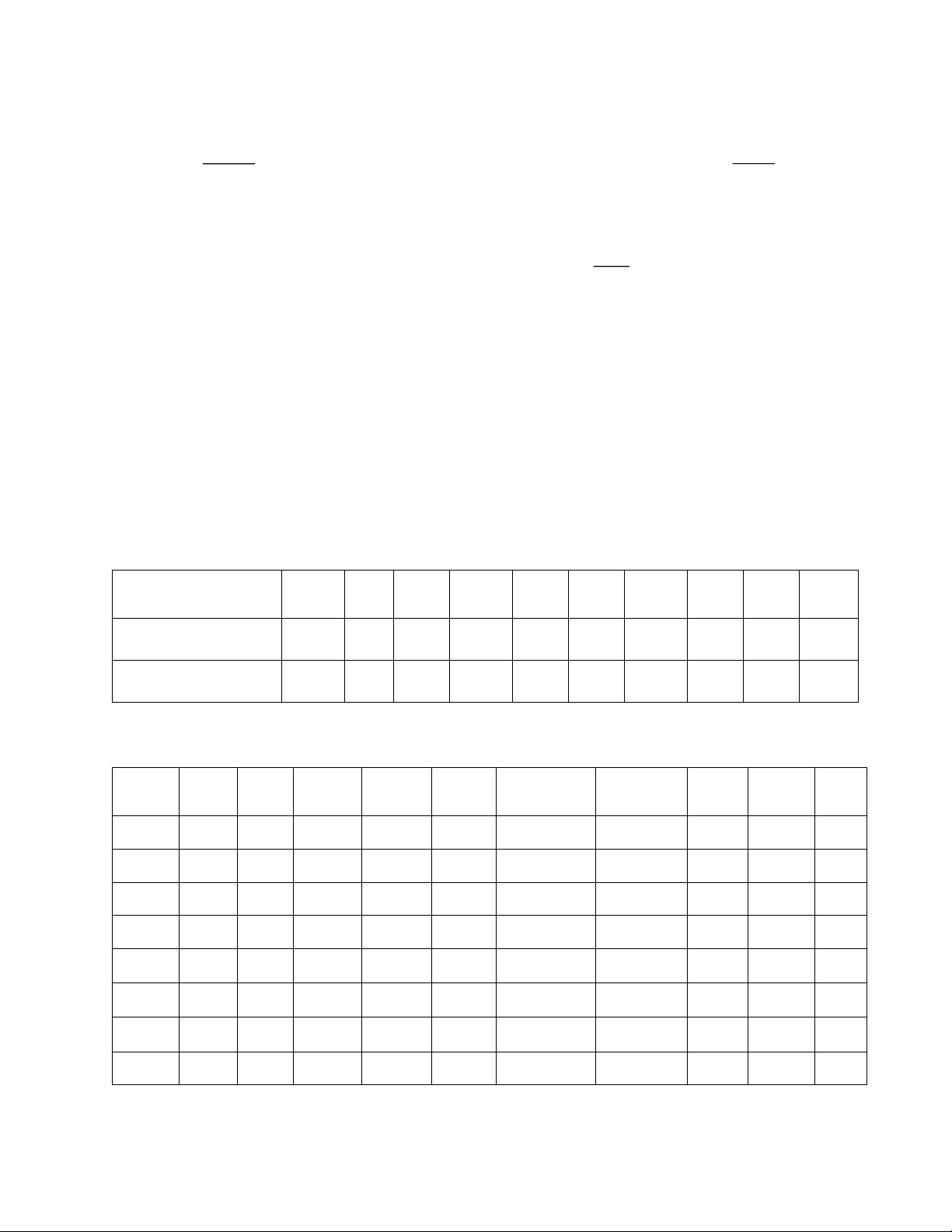1
1
2
1
n
ii
i
n
i
i
xy
b
x
=
=
=
Where
x
is the deviation of X from its mean ( X -
_
X
),
n
X
X
=
_
and
y is deviation of Y from its mean (Y-
_
Y
)
_
Y
Y
n
=
0
b =
_
1
Yb
_
X
Example 1
The following data relates to the sales and profit of ABC Company limited over 10 years. Regress
Profit against sales. Note that from economic theory profits depend on sales. Therefore, sales=X
and profit =Y
Therefore, sales=X and profit =Y
Time
X
Y
XY
X
2
Y
2
_
XXx =
_
YYy =
xy
x
2
y
2
1
10
2
20
100
4
-45
-7
315
2025
49
2
20
3
60
400
9
-35
-6
210
1225
36
3
30
5
150
900
25
-25
-4
100
625
16
4
40
7
280
1600
49
-15
-2
30
225
4
5
50
8
400
2500
64
-5
-1
5
25
1
6
60
9
540
3600
81
5
0
0
25
0
7
70
11
770
4900
121
15
2
30
225
4
8
80
12
960
6400
144
25
3
75
625
9
Time in years
1
2
3
4
5
6
7
8
9
10
Sales (000)
10
20
30
40
50
60
70
80
90
100
Profit (000)
2
3
5
7
8
9
11
12
14
19
Showing Page:
7/9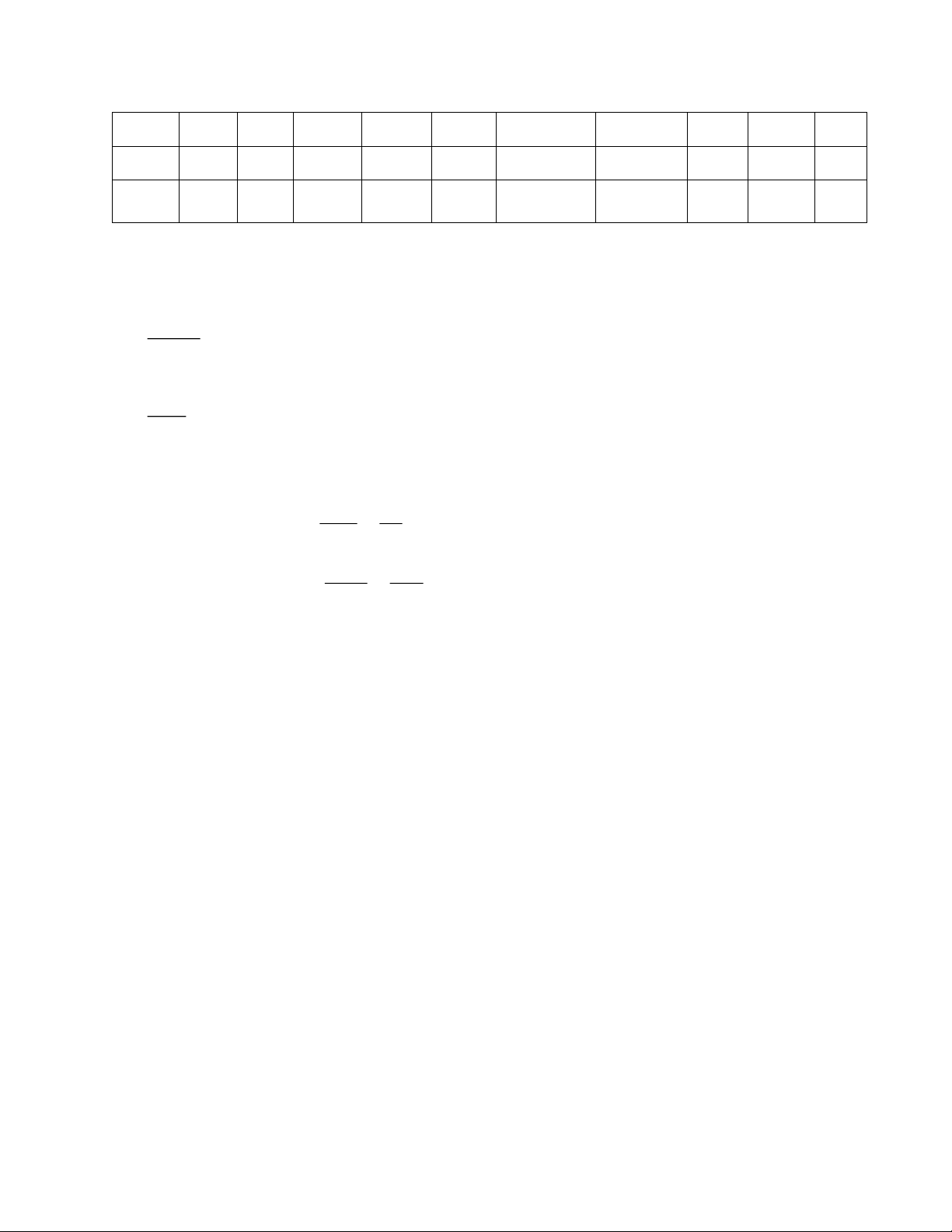9
90
14
1260
8100
196
35
5
175
1225
25
10
100
19
1900
10000
361
45
10
450
2025
100
550
90
6,340
38,500
1,054
1,390
8,250
244
From the regression formula above it implies that;
1
1
2
1
1
1
1390
8250
0.1685
n
ii
i
n
i
i
xy
b
x
b
b
=
=
=
=
=
For
__
01
b Y b X=−
but
55
10
550
9
10
90
_
===
===
n
X
X
n
Y
Y
( )
0
0
0
9 0.1685 55
9 9.2667 0.2667
0.2667
b
b
b
=
= =
=−
Thus the OLS regression Equation is
XY 1685.02667.0 +=
ie Equation of the line of best
fit
Interpretation of the results:
0
0.2667b =−
Assuming sales (x) is zero, the expected or mean profit (Y) is ksh-0.2667 *1000 = sh 266.7(a loss)
1
0.1685b =
An increase in sales by one unit, will lead to an increase in profit by 0.1685 units, ceteris paribus
From the OLS regression equation, we can also predict or forecast the value of Y for any
given value of X.
For example, given that X= 150, we can now predict Y as follows:
Showing Page:
8/9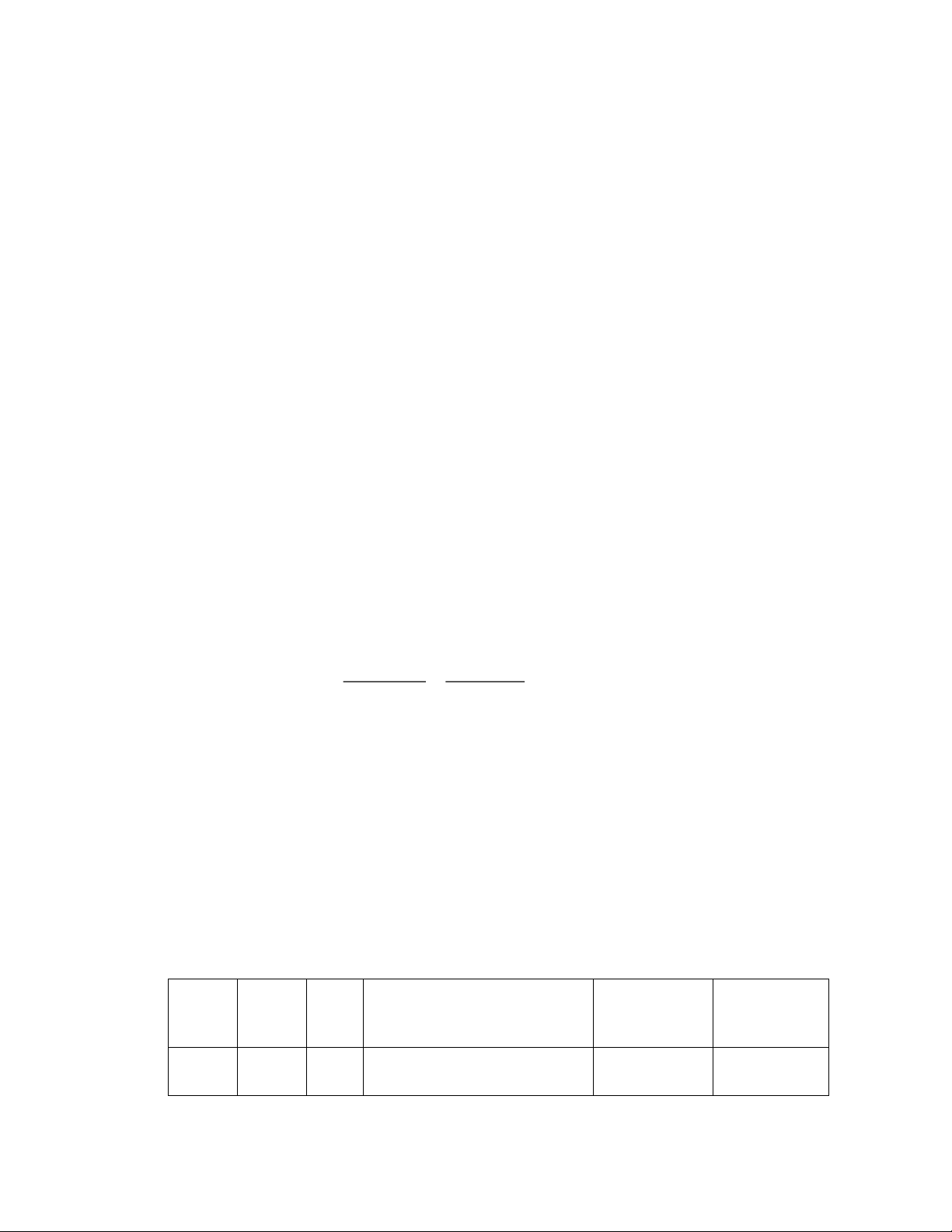XY 1685.02667.0 +=
)150(1685.02667.0 +=
Y
25=
Y
Goodness of Fit (r
2
)
It is also possible to determine the explanatory power (goodness of fit) of the regression
model constructed above.
Goodness of fit gives the proportion of changes in the dependent variable that can be
explained or attributed to changes in all independent variables in the model. For the above
example, the percentage of changes in profit that can be explained by changes in sales.
The remaining proportion (1-r
2
) of changes in profit is explained by other factors.
Goodness of fit (r
2
) can simply be derived by getting Pearson correlation coefficient and
then squaring it. Ie r
2
=
( )
2
2
22
1390
0.9598
244*8250
xy
xy
==

= 95.98%
It can be interpreted to mean that 95.98% of changes in profit can be explained or attributed
to changes in sales. The remaining 4.02% of changes in profitability can be attributed to
other factors influencing profits.
Finally, we can also obtain
Y
( i.e. The estimated value of profits), the residual (
i
e
) and
the squared residuals (
2
i
e
Time
X
Y
XY 1685.02667.0 +=
= YYe
i
2
i
e
1
10
2
1.4183
0.5817
0.3384
Showing Page:
9/9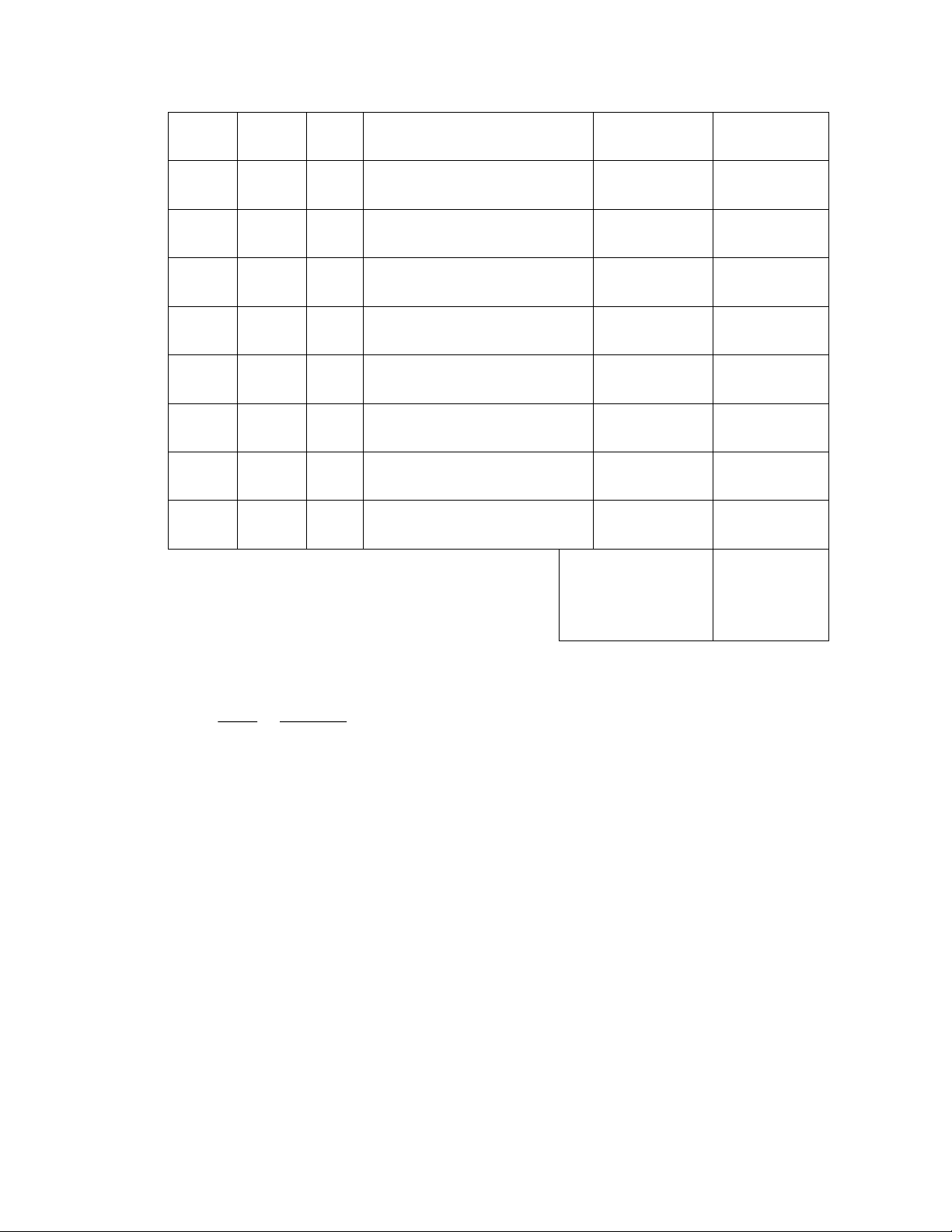2
20
3
3.1033
-0.1033
0.0107
3
30
5
4.7883
0.2117
0.0448
4
40
7
6.4733
0.5267
0.2774
5
50
8
8.1583
-0.1583
0.0251
6
60
9
9.8433
-0.8433
0.7111
7
70
11
11.5283
-0.5283
0.2791
8
80
12
13.2133
-1.2133
1.4721
9
90
14
14.8933
-0.8933
0.8069
10
100
19
16.5833
2.4167
5.8404
= 008.0
i
e
2
i
e
=9.806
Thus, the expected value or mean of the error term is
i
e
is determined as follows:
( )
0008.0
10
008.0
_
=
===
n
e
eEe
i
i
i
Actually, when using OLS, the expected value or mean of the error term should be zero. In
this case, it is not exactly zero due to the rounding off.
Thus E(
i
e
)= Zero (0)
The value 9.806 is called the sum of squared errors/residuals, i.e.
= )(806.9
2
i
.
It can also be calculated as follows:
2 2 2 2
1i
e y b x=−
It implies that,
22
244 (0.16848484 *8250) 9.80608
i
e = =

### Unformatted Attachment Preview

REGRESSION ANALYSIS • . Regression analysis is concerned with the study of the dependence of one variable (the Dependent variable) on one or more other variables (the Explanatory Variable(s) with a view to estimating and/ or predicting the average value of the dependent variable. • Regression analysis aims at finding the equation of the line of best fit in relationship between dependent variable and independent variables. The idea of the line of best fit is derived from the fact that the relationship between Y and X may not be depicted by a straight line such that all coordinates of X and Y falls on that line. • The line of best fit therefore is the line that passes through the points capturing the relationship between dependent and independent variables the best way compared to any other line. • Regression Analysis therefore enable us to come up with mathematical equation of this line of best fit. In case there is linear relationship between X and Y, the equation of line of best fit or regression line will be given as follows: • Ŷ = b0 + b1 X , such that: Yˆ ≡ refer to the estimated values of Y on the line of best fit for any value of X X≡ explanatory variable whose values are usually assume to be fixed or given. b0 = intercept b1 = slope or gradient . Y . . Yˆi = 0 + 1 X i . . . . . X • There are thus two (2) primary differences between correlation and regression analysis, as outlined below: CORRELATION i) Assumes symmetry between X REGRESSION i) Assumes asymmetry between X and Y i.e., there is no distinction and Y; i.e., distinguishes which as to which variable is dependent variable is dependent and which (causality is not important) is explanatory (causality is important) ii) Both X and Y are assumed to be statistical, random or stochastic ii) Only Y is assumed to be statistical but X is assumed to be fixed. Thus, correlation does not imply causality, but regression does so. • There are basically two types of regression analysis, i.e., i) Simple regression analysis ii) Multiple regression analysis • In simple regression analysis, we study the effect of only one explanatory variable on the dependent variable. For example, how X affects Y. Thus, Y=F(X) For this reason, simple regression analysis is also known bivariate regression. In multiple regression analysis, we study the effect of more than one explanatory variable • on the dependent variable. For example, how changes in X1, X2 and X3 will affect Y in: Thus Y=F(X1, X2, …………, Xn). Simple Regression Analysis In performing regression analysis, we can use either the population or a sample data. • The population regression model is given by Yi = 0 + 1 X i + i • The sample regression is given by: Y = b0 + b1 X i + ei Where: - Yi is the actual value of dependent variable ( for the population or sample) - Xi is the independent variable ➢  0 and b0 are the intercept terms of the population sample regression models respectively. ➢  1 and b1 are the slopes or partial derivative of the population regression models respectively i.e. Yi = 1 or b1 as the case may be. X i  ➢ u i is the error term of the population regression model ( (Yi − Y i )  ➢ ei is the error term of the sample regression model (Yi − Y i ) Population Regression . . . .-+U . Y =  +  X u . . . -U . . . Y = + X + .  Y i i . 0 0 1 i 1 i i x Sample Regression . . y . . +e . Y = b + b X . . -e . . . . . Y = b +b X +e  i . • i 0 0 1 1 i i i x Thus, the error is the difference between each observation Y and the estimated value of Y  on the regression line ( Y ) for any given value of X  While Y i = b0 + b1 X i is the equation of the line of best fit, Yi = b0 + b1 X i + ei give coordinate of any point in the data • In regression analysis, we prefer using a sample rather than the population data. This is because, in real life or practice, it is easier to observe a sample data as compared to observing population data. We then use sample regression intercept and slope b0 and b1 as estimators of unknown population parameters ( 0 and 1 ) The Ordinary Least Squares (OLS) Estimation • The Ordinary Least Squares (OLS) Estimation is the main techniques used to estimate regression models/equations. • The name OLS is derived from the fact that this method aims at getting regression coefficients b0 and b1 by minimizing the sum of squared errors. ✓ To illustrate, consider the following sample regression model; Yi = b0 + b1 X i + ei ✓ From this model let’s make error (ei) to be the subject of the above equation as follows; ✓ ei = Yi − b0 − b1 X i ✓ OLS require that we square the error and then sum up all the errors in square form ie n n  e =  (Y − b i =1 2 i i =1 i 0 − b1 X i ) 2 ✓ The last step to get the estimates of b0 and b1 by minimizing e 2 i with respect to the two coefficients. This is by getting partial derivative and then equate to zero   ei2 =0  b0   ei2 and = 0 . We then solve the resulting two equations simultaneously. The  b1 resulting values of b0 and b1 are the one that correspond to the line of best fit. • The ordinary least squares estimators ( b0 and b1 ) are called so because they are used as estimators of unknown population regression parameters B0 and B1 . • As noted above OLS technique has several steps but in summary these two estimators are derived use the following formulas: n • b1 = x y i =1 n i i 2 i _ _ Y= y is deviation of Y from its mean (Y- Y ) • _ X= Where x is the deviation of X from its mean ( X - X ), x i =1 _ _ X and n Y n _ b0 = Y − b1 X Example 1 The following data relates to the sales and profit of ABC Company limited over 10 years. Regress Profit against sales. Note that from economic theory profits depend on sales. Therefore, sales=X and profit =Y Time in years 1 2 3 4 5 6 7 8 9 10 Sales (000) 10 20 30 40 50 60 70 80 90 100 Profit (000) 2 3 5 7 8 9 11 12 14 19 Therefore, sales=X and profit =Y Time X Y XY X2 Y2 x=X −X y = Y −Y xy x2 y2 1 10 2 20 100 4 -45 -7 315 2025 49 2 20 3 60 400 9 -35 -6 210 1225 36 3 30 5 150 900 25 -25 -4 100 625 16 4 40 7 280 1600 49 -15 -2 30 225 4 5 50 8 400 2500 64 -5 -1 5 25 1 6 60 9 540 3600 81 5 0 0 25 0 7 70 11 770 4900 121 15 2 30 225 4 8 80 12 960 6400 144 25 3 75 625 9 _ _ 9 90 14 1260 8100 196 35 5 175 1225 25 10 100 19 1900 10000 361 45 10 450 2025 100  550 90 6,340 38,500 1,054 1,390 8,250 244 From the regression formula above it implies that; n b1 = x y i i =1 n i x i =1 2 i 1390 b1 = 8250 b1 = 0.1685 Y 90 =9 _ _ n 10 For b0 = Y − b1 X but −  X = 550 = 55 X= n 10 _ Y= = b0 = 9 − ( 0.1685  55) b0 = 9 − 9.2667 = −0.2667 b0 = −0.2667  Thus the OLS regression Equation is Y = −0.2667 + 0.1685 X ie Equation of the line of best fit • Interpretation of the results: b0 = −0.2667 Assuming sales (x) is zero, the expected or mean profit (Y) is ksh-0.2667 *1000 = sh 266.7(a loss) b1 = 0.1685 An increase in sales by one unit, will lead to an increase in profit by 0.1685 units, ceteris paribus • From the OLS regression equation, we can also predict or forecast the value of Y for any given value of X. For example, given that X= 150, we can now predict Y as follows:  Y = −0.2667 + 0.1685 X  Y = −0.2667 + 0.1685(150)  Y = 25 Goodness of Fit (r2) • It is also possible to determine the explanatory power (goodness of fit) of the regression model constructed above. • Goodness of fit gives the proportion of changes in the dependent variable that can be explained or attributed to changes in all independent variables in the model. For the above example, the percentage of changes in profit that can be explained by changes in sales. • The remaining proportion (1-r2) of changes in profit is explained by other factors. • Goodness of fit (r2) can simply be derived by getting Pearson correlation coefficient and  ( xy ) x y 2 2 then squaring it. Ie r = • 2 2 = 13902 = 0.9598 = 95.98% 244*8250 It can be interpreted to mean that 95.98% of changes in profit can be explained or attributed to changes in sales. The remaining 4.02% of changes in profitability can be attributed to other factors influencing profits. •  Finally, we can also obtain Y ( i.e. The estimated value of profits), the residual ( ei ) and the squared residuals ( e i 2 ) or RSS as follows:   2 Time X Y Y = −0.2667 + 0.1685 X ei = Y − Y ei 1 10 2 1.4183 0.5817 0.3384 2 20 3 3.1033 -0.1033 0.0107 3 30 5 4.7883 0.2117 0.0448 4 40 7 6.4733 0.5267 0.2774 5 50 8 8.1583 -0.1583 0.0251 6 60 9 9.8433 -0.8433 0.7111 7 70 11 11.5283 -0.5283 0.2791 8 80 12 13.2133 -1.2133 1.4721 9 90 14 14.8933 -0.8933 0.8069 10 100 19 16.5833 2.4167 5.8404 e i = −0.008 e 2 i =9.806 • Thus, the expected value or mean of the error term is ei is determined as follows: e i = E (ei ) = _ • e = i n − 0.008 = −0.0008 10 Actually, when using OLS, the expected value or mean of the error term should be zero. In this case, it is not exactly zero due to the rounding off. Thus E( ei )= Zero (0) • The value 9.806 is called the sum of squared errors/residuals, i.e.  ei = 9.806( RSS) . 2 It can also be calculated as follows: It implies that, e i 2 e i 2 =  y 2 − b12  x 2 = 244 − (0.168484842 *8250) = 9.80608 Name: Description: ...User generated content is uploaded by users for the purposes of learning and should be used following Studypool's honor code & terms of service.Studypool4.7Trustpilot4.5Sitejabber4.4